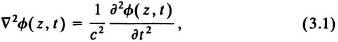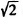# Physical Optics

## 3.1.    INTRODUCTION

The basic theory of geometrical optics as given in Chapter 2 describes how optical elements such as lenses, mirrors, and prisms modify the direction of light rays. Physical optics extends the theoretical treatment of optical systems by incorporating the wave nature of light. We take a direct approach and refer the reader to various texts that provide the details of the development from Maxwell’s equation (10, 1518).

We begin with the basic assumption that light waves propagate in an isotropic media with simple harmonic motion and satisfy the scalar wave equationwhere t is time and z is distance in the direction that the wave travels. The representation for free-space electromagnetic radiation is a real-valued function of the form cos(ωtkz), where k = 2π/λ and ω is the radian frequency of light.

The transfer functions for lenses, prisms, and other optical elements are usually represented by complex-valued functions. As an example, Figure 3.1 shows an arbitrary optical element, illuminated by monochromatic light at wavelength λ that propagates parallel to the z axis. The light wave at plane z0, represented bycos(ωtkz0), is spatially modulated by the optical element whose magnitude transmittance is |a(x)| and whose phase is (x). The phase ...

Get Optical Signal Processing now with the O’Reilly learning platform.

O’Reilly members experience books, live events, courses curated by job role, and more from O’Reilly and nearly 200 top publishers.